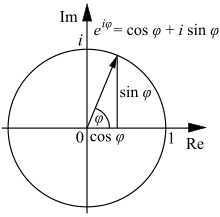# 2 complex number questions

How do you get the exponential form of 1/j? I saw a problem that says it's e^(-j*pi/2) but I have no idea where that came from.

Also, if you have a complex number, z, how do you find it's magnitude? For example, e^(j*pi*t - pi/2). In my book when they square the the magnitude of a complex number they multiply by the conjugate (e.g. |z|^2 = e^(j*pi*t - pi/2) * e^(-j*pi*t + pi/2) Why is this? Thanks for any help.

LeonhardEuler
Gold Member
For your first question, note that 1/j = -j. To get this take 1/j and multiply by j/j:
$$\frac{1}{j}=\frac{j}{j^2}=-j$$

For your second question, the definition of the magnitude of a complex number is that it is the square root of itself times its complex conjugate. To see that this corresponds to the distance from the origin when complex numbers are plotted as points in a plane, notice that if z=a+bj, then
zz*=(a+bj)(a-bj)=a2 + b2
Which is the square of the distance from the origin by the Pythagorean theorem.

How do you get the exponential form of 1/j? I saw a problem that says it's e^(-j*pi/2) but I have no idea where that came from.

$$e^{i \pi} + 1 = 0$$

==>

$$e^{i \pi} = -1$$

$$(e^{i \pi})^{1/2} = \sqrt{-1} = i$$

$$e^{i \pi/2} = i$$

$$(e^{i \pi/2})^{-1} = \frac{1}{i} = -i$$

$$e^{-i \pi/2} = -i$$

$$e^{i\varphi} = \cos{\varphi} + i \sin{\varphi}$$

http://en.wikipedia.org/wiki/Euler's_formula

Now from this Wikipedia diagram of the complex plane...... it can be seen that when the real part is zero, that $\varphi$ must be $\pi/2$ so:

$$e^{i\pi/2} = \cos{(\pi/2)} + i \sin{(\pi/2)} = i$$

(since $\cos{(\pi/2)}=0$ and $\sin{(\pi/2)}=1$)

$$e^{-i\pi/2} = -i = 1/i$$

Last edited by a moderator:
ohhh my god... that question make me headache almost the time.....:)

chwala
Gold Member
if z is a complex no...Z=X =iY then the magnitude of Z is simply =√ X+iY

HallsofIvy
Homework Helper
if z is a complex no...Z=X =iY then the magnitude of Z is simply =√ X+iY
Completely wrong. If Z= X+iY then the magnitude of Z is the non-negative real number
$$\sqrt{X^2+ Y^2}$$

$$\sqrt{X+ iY}$$
is any of those complex numbers whose square is X+ iY

For example, if z= i= 0+ i(1) then the magnitude of z is $|z|= \sqrt{1^2+ 1^2}= \sqrt{2}$. But
$$\sqrt{z}= \sqrt{ i}= \frac{\sqrt{2}}{2}+ \frac{\sqrt{2}}{2}i$$
or
$$\sqrt{z}= \sqrt{i}= \frac{\sqrt{2}}{2}- \frac{\sqrt{2}}{2}i$$